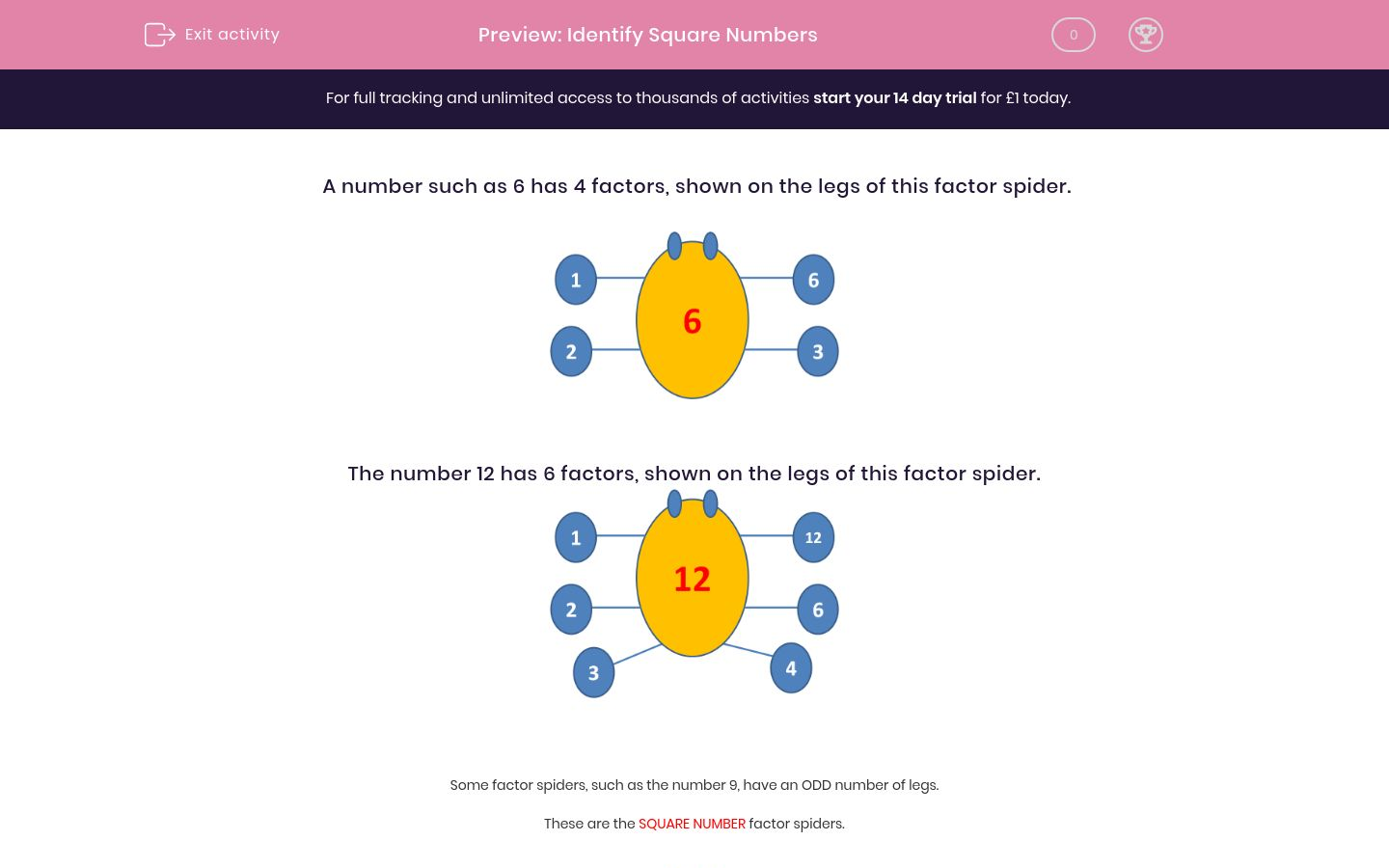# Identify Square Numbers

In this worksheet, children must state whether or not a number is square.Key stage:  KS 2

Curriculum topic:   Maths and Numerical Reasoning

Curriculum subtopic:   Factors and Multiples

Difficulty level:### QUESTION 1 of 10

A number such as 6 has 4 factors, shown on the legs of this factor spider.The number 12 has 6 factors, shown on the legs of this factor spider.Some factor spiders, such as the number 9, have an ODD number of legs.

These are the SQUARE NUMBER factor spiders.Remember that 9 = 3 x 3 = 3 squared.

---- OR ----